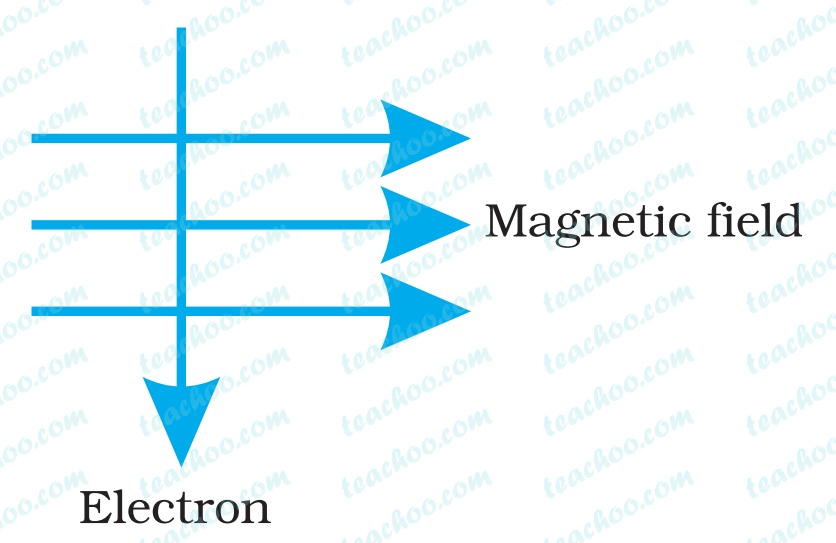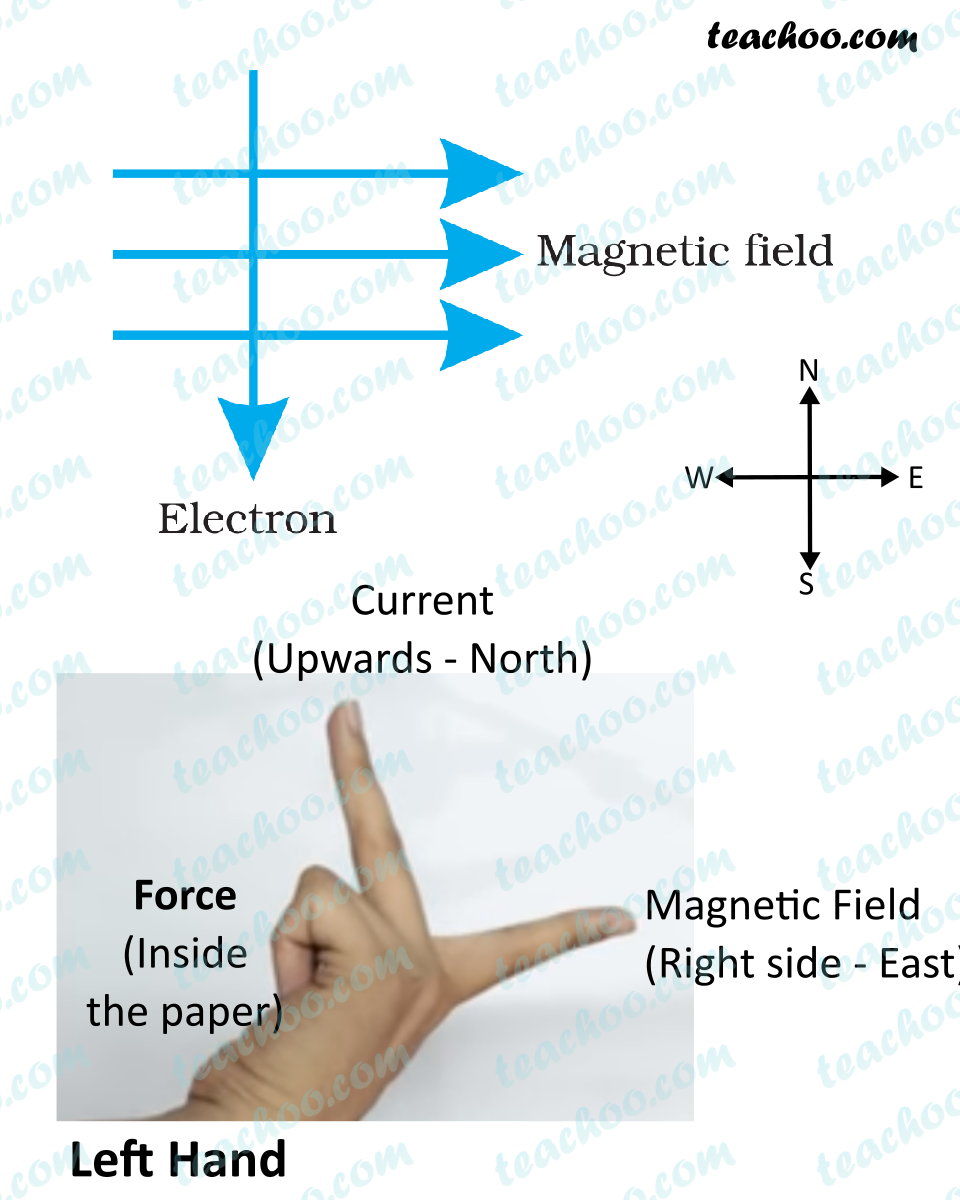## An electron enters a magnetic field at right angles to it, as shown in Fig. 13.14. The direction of force acting on the electron will be

(a) to the right.

(b) to the left.

(c) out of the page.

(d) into the page.The correct answer is (d) Into the plane

When electron moves downwards,

i.e. Negatively charged particle moves downwards

So, Direction of current is upwards (towards North)

And, Magnetic field is from West to East (Left to Right )Applying Fleming’s left hand rule,

Taking the forefinger, middle finger and thumb of the left hand in mutually perpendicular direction

• Pointing middle finger towards north direction (direction of current)
• Fore-finger towards east/right direction (direction of magnetic field)
• We get the direction of force is into the plane , since the thumb points into the plane.

So, direction of force for both electron is Into the plane

1. Class 10
2. Chapter 13 Class 10 - Magnetic Effects of Electric Current (Term 2)
3. Examples from NCERT Book

Examples from NCERT Book

Class 10
Chapter 13 Class 10 - Magnetic Effects of Electric Current (Term 2)